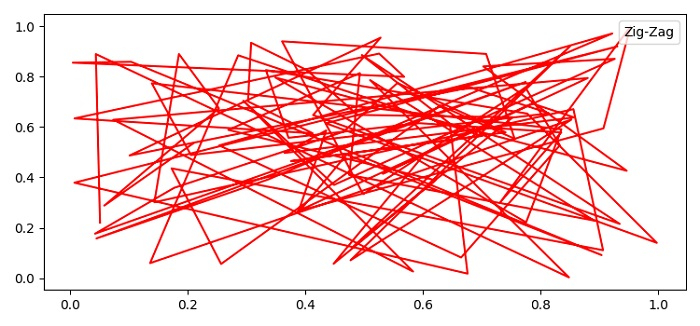# How do you just show the text label in a plot legend in Matplotlib?

To just show the text label in plot legend we can use legend method with handlelength=0, handletextpad=0 and fancybox=0 in the arguments.

## Steps

• Set the figure size and adjust the padding between and around the subplots.

• Create random x and y data points using numpy.

• Create a figure and a set of subplots using subplots() method.

• Plot x and y data points using plot() method with label "Zig-Zag" for the legend.

• Use legend() method to place the label for the plot with handlelength=0, handletextpad=0 and fancybox=0 in the arguments.

• To display the figure, use show() method.

## Example

import numpy as np
from matplotlib import pyplot as plt
plt.rcParams["figure.figsize"] = [7.50, 3.50]
plt.rcParams["figure.autolayout"] = True
x = np.random.rand(100)
y = np.random.rand(100)
fig, ax = plt.subplots()
ax.plot(x, y, label='Zig-Zag', c="red")
plt.show()